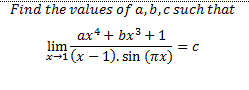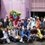A limit problemI have a problem in limit that made me curious until now. The problem is about the limit and trigonometry. I don't know how to simplify it.. pls help me to solve it. thanks..:)Note by Leonardo Chandra
6 years, 4 months ago

This discussion board is a place to discuss our Daily Challenges and the math and science related to those challenges. Explanations are more than just a solution — they should explain the steps and thinking strategies that you used to obtain the solution. Comments should further the discussion of math and science.

When posting on Brilliant:

• Use the emojis to react to an explanation, whether you're congratulating a job well done , or just really confused .
• Ask specific questions about the challenge or the steps in somebody's explanation. Well-posed questions can add a lot to the discussion, but posting "I don't understand!" doesn't help anyone.
• Try to contribute something new to the discussion, whether it is an extension, generalization or other idea related to the challenge.
• Stay on topic — we're all here to learn more about math and science, not to hear about your favorite get-rich-quick scheme or current world events.

MarkdownAppears as
*italics* or _italics_ italics
**bold** or __bold__ bold

- bulleted
- list

• bulleted
• list

1. numbered
2. list

1. numbered
2. list
Note: you must add a full line of space before and after lists for them to show up correctly
paragraph 1

paragraph 2

paragraph 1

paragraph 2

> This is a quote
This is a quote
# I indented these lines
# 4 spaces, and now they show
# up as a code block.

print "hello world"
# I indented these lines
# 4 spaces, and now they show
# up as a code block.

print "hello world"
MathAppears as
Remember to wrap math in $$...$$ or $...$ to ensure proper formatting.
2 \times 3 $2 \times 3$
2^{34} $2^{34}$
a_{i-1} $a_{i-1}$
\frac{2}{3} $\frac{2}{3}$
\sqrt{2} $\sqrt{2}$
\sum_{i=1}^3 $\sum_{i=1}^3$
\sin \theta $\sin \theta$
\boxed{123} $\boxed{123}$

Sort by:

I assume $c$ is a finite value

The key here is L'hôpital's Rule (3 times)

Substitution of $x=1$ must give an indeterminate form of $\frac {0}{0}$ because denominator yields $0$

So the numerator is equals to $0$ when $x=1 \Rightarrow a+b+1=0$

Apply L'hôpital's Rule and chain rule, the limit becomes

$\frac {4 a x^{3} + 3 b x^{2}} { \pi (x - 1) \cos (\pi x) + \sin (\pi x) }$

And it must yield an indeterminate form of $\frac {0}{0}$ as well because denominator yields $0$ at $x=1$, thus numerator must yield $0$ as well

So, $4a+3b=0$, solve the two equations gives $a = 3$, $b=-4$

Now substitute those two values into the limit and apply L'hôpital's Rule Rule once again

$\frac {36 x^{2} - 24 x } { \pi [ -(x-1) \pi \sin (\pi x) + \cos (\pi x) ] + \pi \cos (\pi x) }$

Substitute $x=1$ gives $c = - \frac {6}{\pi}$

Hence, $a = 3, b=-4, c = - \frac {6}{\pi}$

By the way, I'm new to LaTeX, anyone know how to increase size of the mathematical symbols used? The fractions displayed are too small in standard font size.

- 6 years, 4 months ago

yeah agreed......with the answer...:)

- 6 years, 4 months ago

thanks for the answer, Pi Han..I never consider the using of 0/0 to evaluate the value of numerator, then apply L'hopital more than twice to find the answer. Anyway, thanks for the great answer..I need to learn more again about the L'Hopital rule. :)

- 6 years, 4 months ago

from where did u get this problem?

- 6 years, 4 months ago

I got this problem from my calculus book. this problem is about limit problems..an introduction to improper form of limit..:)

- 6 years, 4 months ago

How about We asume that limit when $x \rightarrow 1$ is 0/0. Then, calculate it using L'Hopital Theorem.. Sorry, I haven't tried that before..

- 6 years, 4 months ago

since denominator at x=1 tends to 0 then for the limit to exist ,numerator has to be 0 or else it will form indeterminate form....

0/0 is a basic form in limits....:)

- 6 years, 4 months ago

thank you very much for explanation :)

- 6 years, 4 months ago

no problem....

- 6 years, 4 months ago